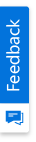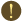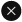We use cookies to give you the best experience on our website. If you continue to browse, then you agree to our privacy policy and cookie policy.Unfortunately, activation email could not send to your email. Please try again.# How to handle "Index Out of Range Exception" while accessing first Excel worksheet?

Platform: WinForms |
Control: XlsIO

Syncfusion Excel (XlsIO) library is a .NET Excel library used to create, read, and edit Excel documents. Also, converts Excel documents to PDF files.

## Why does the Index Out of Range Exception is thrown?

Index Out of Range exception occurs when wrong index value is specified. For example, if an Excel workbook has 3 worksheets then their respective indices will be 0,1 and 2. Trying to access the worksheet with index 3 and above results in Index Out of Range exception.

## How to overcome Index Out of Range Exception?

Index must always be non-negative and less than the size of collection. The worksheet index in an Excel workbook starts with 0.

The below sample explains how to access the first worksheet in an Excel workbook without facing Index Out of Range exception.

C#

```using (ExcelEngine excelEngine = new ExcelEngine())
{
//Instantiate the Excel application object
IApplication application = excelEngine.Excel;

//Set the default application version
application.DefaultVersion = ExcelVersion.Xlsx;

//Create a new Excel workbook
IWorkbook workbook = application.Workbooks.Create();

//Workbook will be created with 3 worksheets by default, with indices as 0,1 and 2 respectively
//Get the first worksheet in the workbook into IWorksheet and assign some text in a cell
IWorksheet worksheet = workbook.Worksheets;
worksheet.Range["A1"].Text = "First worksheet";

//Get the second worksheet in the workbook into IWorksheet and assign some text in a cell
worksheet = workbook.Worksheets;
worksheet.Range["A1"].Text = "Second worksheet";

//Get the third worksheet in the workbook into IWorksheet and assign some text in a cell
worksheet = workbook.Worksheets;
worksheet.Range["A1"].Text = "Third worksheet";

//Save the Excel workbook
workbook.SaveAs("Output.xlsx");
}
```

VB.NET

```Using excelEngine As ExcelEngine = New ExcelEngine()
'Instantiate the Excel application object
Dim application As IApplication = excelEngine.Excel

'Set the default application version
application.DefaultVersion = ExcelVersion.Xlsx

'Create a new Excel workbook
Dim workbook As IWorkbook = application.Workbooks.Create()

'Workbook will be created with 3 worksheets by default, with indices as 0,1 and 2 respectively
'Get the first worksheet in the workbook into IWorksheet and assign some text in a cell
Dim worksheet As IWorksheet = workbook.Worksheets(0)
worksheet.Range("A1").Text = "First worksheet"

'Get the second worksheet in the workbook into IWorksheet and assign some text in a cell
worksheet = workbook.Worksheets(1)
worksheet.Range("A1").Text = "Second worksheet"

'Get the third worksheet in the workbook into IWorksheet and assign some text in a cell
worksheet = workbook.Worksheets(2)
worksheet.Range("A1").Text = "Third worksheet"

'Save the Excel workbook
workbook.SaveAs("Output.xlsx")
End Using
```

A complete working sample to access the first worksheet in an Excel workbook without facing the Index Out of Range exception can be downloaded from Exception-Handling.zip.

Take a moment to peruse the documentation, where you can find basic worksheet data manipulation options along with features like Conditional Formatting, worksheet calculations through Formulas, adding Charts in worksheet or workbook, organizing and analyzing data through Tables and Pivot Tables, appending multiple records to worksheet using Template Markers, and most importantly PDF and Image conversions etc., with code examples.

Click here to explore the rich set of Syncfusion Excel (XlsIO) library features.

Note:

Starting with v16.2.0.x, if you reference Syncfusion assemblies from trial setup or from the NuGet feed, include a license key in your projects. Refer the link to learn about generating and registering Syncfusion license key in your application to use the components without trail message.

2X faster development

The ultimate WinForms UI toolkit to boost your development speed.You are using an outdated version of Internet Explorer that may not display all features of this and other websites. Upgrade to Internet Explorer 8 or newer for a better experience.